Test: Probability Theory - 2

# Test: Probability Theory - 2 - SSC CGL

Test Description

## 10 Questions MCQ Test Statistics for SSC CGL - Test: Probability Theory - 2

Test: Probability Theory - 2 for SSC CGL 2023 is part of Statistics for SSC CGL preparation. The Test: Probability Theory - 2 questions and answers have been prepared according to the SSC CGL exam syllabus.The Test: Probability Theory - 2 MCQs are made for SSC CGL 2023 Exam. Find important definitions, questions, notes, meanings, examples, exercises, MCQs and online tests for Test: Probability Theory - 2 below.
Solutions of Test: Probability Theory - 2 questions in English are available as part of our Statistics for SSC CGL for SSC CGL & Test: Probability Theory - 2 solutions in Hindi for Statistics for SSC CGL course. Download more important topics, notes, lectures and mock test series for SSC CGL Exam by signing up for free. Attempt Test: Probability Theory - 2 | 10 questions in 10 minutes | Mock test for SSC CGL preparation | Free important questions MCQ to study Statistics for SSC CGL for SSC CGL Exam | Download free PDF with solutions
 1 Crore+ students have signed up on EduRev. Have you?
Test: Probability Theory - 2 - Question 1

### Tickets numbered 1 to 20 are mixed up and then a ticket is drawn at random. What is the probability that the ticket drawn has a number which is a multiple of 3 or 5?

Detailed Solution for Test: Probability Theory - 2 - Question 1

Here, S = {1, 2, 3, 4, ...., 19, 20}
Let E = event of getting a multiple of 3 or 5
= {3, 6 , 9, 12, 15, 18, 5, 10, 20}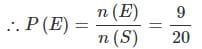Test: Probability Theory - 2 - Question 2

### In a box, there are 8 red, 7 blue and 6 green balls. One ball is picked up randomly. What is the probability that it is neither red nor green?

Detailed Solution for Test: Probability Theory - 2 - Question 2

Total number of balls
= (8 + 7 + 6)
= 21
Let E = event that the ball drawn is neither red nor green
= event that the ball drawn is blue
∴n(E)=7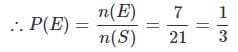Test: Probability Theory - 2 - Question 3

### Three unbiased coins are tossed. What is the probability of getting at most two heads?

Detailed Solution for Test: Probability Theory - 2 - Question 3

Getting at most Two heads means 0 to 2 but not more than 2
Here S = {TTT, TTH, THT, HTT, THH, HTH, HHT, HHH}
Let E = event of getting at most two heads
Then E = {TTT, TTH, THT, HTT, THH, HTH, HHT}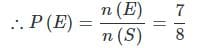Test: Probability Theory - 2 - Question 4

In a class, there are 15 boys and 10 girls. Three students are selected at random. The probability that 1 girl and 2 boys are selected, is:

Detailed Solution for Test: Probability Theory - 2 - Question 4

Let S be the sample space and E be the event of selecting 1 girl and 2 boys
Then, n(S) = Number ways of selecting 3 students out of 25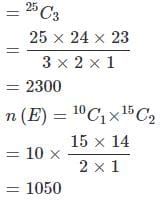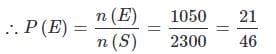Test: Probability Theory - 2 - Question 5

From a pack of 52 cards, two cards are drawn together at random. What is the probability of both the cards being kings?

Detailed Solution for Test: Probability Theory - 2 - Question 5

Let S be the sample space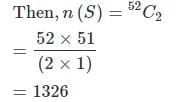Let E = event of getting 2 kings out of 4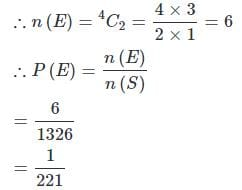Test: Probability Theory - 2 - Question 6

A bag contains 4 white, 5 red and 6 blue balls. Three balls are drawn at random from the bag. The probability that all of them are red, is:

Detailed Solution for Test: Probability Theory - 2 - Question 6

Let S be the sample space
Then, n(S) = number of ways of drawing 3 balls out of 15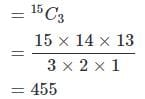Let E = event of getting all the 3 red balls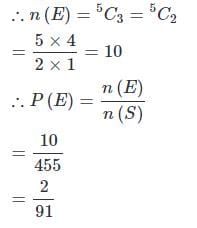Test: Probability Theory - 2 - Question 7

One card is drawn at random from a pack of 52 cards. What is the probability that the card drawn is a face card (Jack, Queen and King only)?

Detailed Solution for Test: Probability Theory - 2 - Question 7

Clearly, there are 52 cards, out of which there are 12 face cards.
∴P(getting a face card)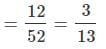Test: Probability Theory - 2 - Question 8

Tickets numbered 1 to 20 are mixed up and then a ticket is drawn at random. What is the probability that the ticket drawn bears a number which is a multiple of 3 ?

Detailed Solution for Test: Probability Theory - 2 - Question 8

Here, S = {1, 2, 3, 4,........, 19, 20}
Let E = even of getting a multiple of 3 = {3, 6, 9, 12, 15, 18}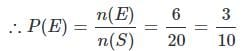Test: Probability Theory - 2 - Question 9

An urn contains 6 red, 4 blue, 2 green and 3 yellow marbles. If 4 marbles are picked up at random, what is the probability that at least one of them is blue?

Detailed Solution for Test: Probability Theory - 2 - Question 9

Total number of marbles = (6 + 4 + 2 + 3) = 15
Let E be the event of drawing 4 marbles such that none is blue.
Then, n(E) = number of ways of drawing 4 marbles out of 11 non-blue.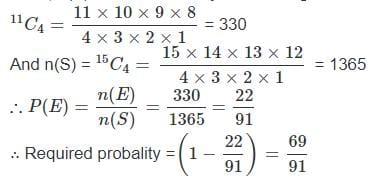Test: Probability Theory - 2 - Question 10

A card is drawn from a pack of 52 cards. The probability of getting a queen of club or a king of heart is-

Detailed Solution for Test: Probability Theory - 2 - Question 10

Hence, n(S) = 52
Let E = event of getting a queen of club or a king of heart.
Then, n(E) = 2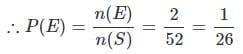## Statistics for SSC CGL

69 videos|90 docs|16 tests
Information about Test: Probability Theory - 2 Page
In this test you can find the Exam questions for Test: Probability Theory - 2 solved & explained in the simplest way possible. Besides giving Questions and answers for Test: Probability Theory - 2, EduRev gives you an ample number of Online tests for practice

## Statistics for SSC CGL

69 videos|90 docs|16 tests